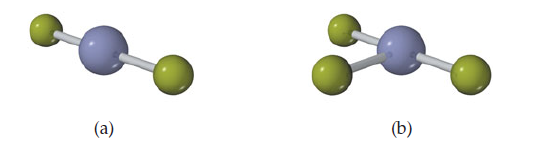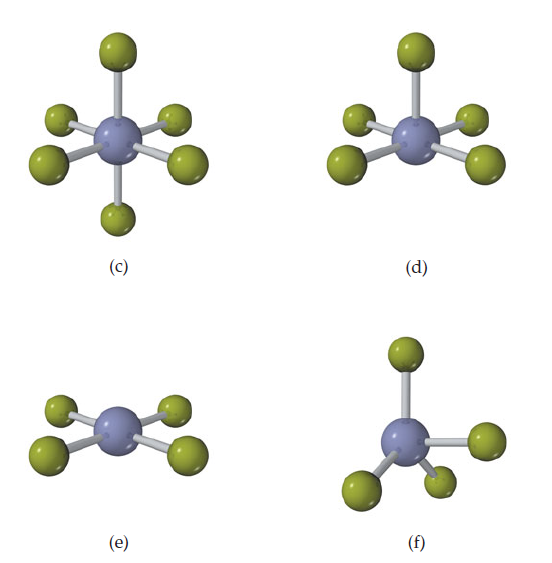×
Get Full Access to Chemistry: The Central Science - 12 Edition - Chapter 9 - Problem 3e
Get Full Access to Chemistry: The Central Science - 12 Edition - Chapter 9 - Problem 3e

×

# Visualizing ConceptsFor each molecule (a)–(f), indicateISBN: 9780321696724 27

## Solution for problem 3E Chapter 9

Chemistry: The Central Science | 12th Edition

• Textbook Solutions
• 2901 Step-by-step solutions solved by professors and subject experts
• Get 24/7 help from StudySoup virtual teaching assistantsChemistry: The Central Science | 12th Edition

4 5 1 432 Reviews
27
2
Problem 3E

For each molecule (a)-(f), indicate how many different electron- domain geometries are consistent with the molecular geometry shown. [Section 9.2]Step-by-Step Solution:
Step 1 of 3

Friday, February 12, 2016 11:24 AM New Section 1 Page 1 New Section 1 Page 2 New Section 1 Page 3 New Section 1 Page 4 Friday, February 19, 2016 11:20 AM New Section 1 Page 1 New Section 1 Page 2 New Section 1 Page 3 New Section 1 Page 4

Step 2 of 3

Step 3 of 3

##### ISBN: 9780321696724

Chemistry: The Central Science was written by and is associated to the ISBN: 9780321696724. The answer to “?For each molecule (a)-(f), indicate how many different electron- domain geometries are consistent with the molecular geometry shown. [Section 9.2]” is broken down into a number of easy to follow steps, and 20 words. Since the solution to 3E from 9 chapter was answered, more than 1087 students have viewed the full step-by-step answer. This textbook survival guide was created for the textbook: Chemistry: The Central Science, edition: 12. This full solution covers the following key subjects: concepts, consistent, domain, electron, Geometries. This expansive textbook survival guide covers 49 chapters, and 5471 solutions. The full step-by-step solution to problem: 3E from chapter: 9 was answered by , our top Chemistry solution expert on 04/03/17, 07:58AM.

## Discover and learn what students are asking

Calculus: Early Transcendental Functions : Partial Derivatives
?In Exercises 7 - 38, find both first partial derivatives. $$z=y e^{y / x}$$

Calculus: Early Transcendental Functions : Extrema of Functions of Two Variables
?In Exercises 7 - 20, examine the function for relative extrema and saddle points. $$f(x, y)=x^{2}-x y-y^{2}-3 x-y$$

Statistics: Informed Decisions Using Data : Scatter Diagrams and Correlation
?True or False: If the linear correlation coefficient is close to 0, then the two variables have no relation.

Unlock Textbook Solution